# US10833613B2 - Inverter control apparatus and motor drive system - Google Patents

## Info

Publication number
US10833613B2
US10833613B2 US16/291,423 US201916291423A US10833613B2 US 10833613 B2 US10833613 B2 US 10833613B2 US 201916291423 A US201916291423 A US 201916291423A US 10833613 B2 US10833613 B2 US 10833613B2
Authority
US
United States
Prior art keywords
electric
current
axis
value
synchronous motor
Prior art date
Legal status (The legal status is an assumption and is not a legal conclusion. Google has not performed a legal analysis and makes no representation as to the accuracy of the status listed.)
Active
Application number
US16/291,423
Other versions
US20190199253A1 (en
Inventor
Tomoaki Shigeta
Shun Taniguchi
Kazuya Yasui
Current Assignee (The listed assignees may be inaccurate. Google has not performed a legal analysis and makes no representation or warranty as to the accuracy of the list.)
Toshiba Corp
Toshiba Infrastructure Systems and Solutions Corp
Original Assignee
Toshiba Corp
Toshiba Infrastructure Systems and Solutions Corp
Priority date (The priority date is an assumption and is not a legal conclusion. Google has not performed a legal analysis and makes no representation as to the accuracy of the date listed.)
Filing date
Publication date
Priority to JP2016173087 priority Critical
Priority to JP2016-173087 priority
Priority to PCT/JP2017/030980 priority patent/WO2018043499A1/en
Application filed by Toshiba Corp, Toshiba Infrastructure Systems and Solutions Corp filed Critical Toshiba Corp
Assigned to TOSHIBA INFRASTRUCTURE SYSTEMS & SOLUTIONS CORPORATION, KABUSHIKI KAISHA TOSHIBA reassignment TOSHIBA INFRASTRUCTURE SYSTEMS & SOLUTIONS CORPORATION ASSIGNMENT OF ASSIGNORS INTEREST (SEE DOCUMENT FOR DETAILS). Assignors: YASUI, KAZUYA, SHIGETA, TOMOAKI
Publication of US20190199253A1 publication Critical patent/US20190199253A1/en
Publication of US10833613B2 publication Critical patent/US10833613B2/en
Application granted granted Critical
Active legal-status Critical Current
Anticipated expiration legal-status Critical

• 230000001360 synchronised Effects 0.000 claims abstract description 125
• 230000003068 static Effects 0.000 claims description 35
• 230000004907 flux Effects 0.000 claims description 10
• 230000014509 gene expression Effects 0.000 description 109
• 238000010586 diagram Methods 0.000 description 28
• 239000000969 carrier Substances 0.000 description 12
• 230000000051 modifying Effects 0.000 description 12
• 230000004044 response Effects 0.000 description 8
• 241001442055 Vipera berus Species 0.000 description 7
• 235000020127 ayran Nutrition 0.000 description 5
• 230000001131 transforming Effects 0.000 description 5
• 230000000694 effects Effects 0.000 description 4
• 238000004804 winding Methods 0.000 description 3
• 230000000052 comparative effect Effects 0.000 description 2
• 230000002596 correlated Effects 0.000 description 2
• 239000000686 essence Substances 0.000 description 2
• 239000000203 mixture Substances 0.000 description 2
• 229910000831 Steel Inorganic materials 0.000 description 1
• 230000004075 alteration Effects 0.000 description 1
• 238000006243 chemical reaction Methods 0.000 description 1
• 229910052802 copper Inorganic materials 0.000 description 1
• 239000010949 copper Substances 0.000 description 1
• 230000003111 delayed Effects 0.000 description 1
• 230000036545 exercise Effects 0.000 description 1
• 239000000284 extract Substances 0.000 description 1
• 238000000034 method Methods 0.000 description 1
• 230000004048 modification Effects 0.000 description 1
• 238000006011 modification reaction Methods 0.000 description 1
• 239000010959 steel Substances 0.000 description 1

## Images

•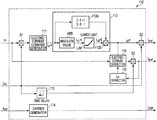•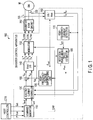•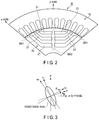••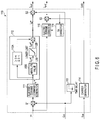•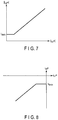••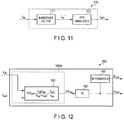•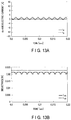•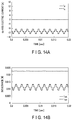•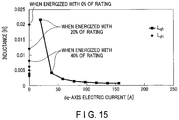•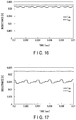•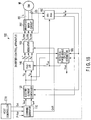•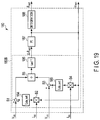•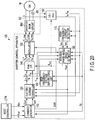•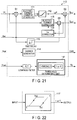•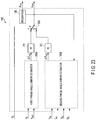•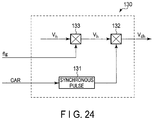•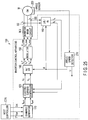•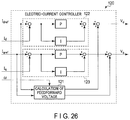•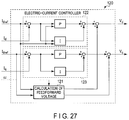## Classifications

• HELECTRICITY
• H02GENERATION; CONVERSION OR DISTRIBUTION OF ELECTRIC POWER
• H02PCONTROL OR REGULATION OF ELECTRIC MOTORS, ELECTRIC GENERATORS OR DYNAMO-ELECTRIC CONVERTERS; CONTROLLING TRANSFORMERS, REACTORS OR CHOKE COILS
• H02P6/00Arrangements for controlling synchronous motors or other dynamo-electric motors using electronic commutation dependent on the rotor position; Electronic commutators therefor
• H02P6/14Electronic commutators
• H02P6/16Circuit arrangements for detecting position
• H02P6/18Circuit arrangements for detecting position without separate position detecting elements
• H02P6/181Circuit arrangements for detecting position without separate position detecting elements using different methods depending on the speed
• HELECTRICITY
• H02GENERATION; CONVERSION OR DISTRIBUTION OF ELECTRIC POWER
• H02PCONTROL OR REGULATION OF ELECTRIC MOTORS, ELECTRIC GENERATORS OR DYNAMO-ELECTRIC CONVERTERS; CONTROLLING TRANSFORMERS, REACTORS OR CHOKE COILS
• H02P21/00Arrangements or methods for the control of electric machines by vector control, e.g. by control of field orientation
• H02P21/14Estimation or adaptation of machine parameters, e.g. flux, current or voltage
• H02P21/141Flux estimation
• HELECTRICITY
• H02GENERATION; CONVERSION OR DISTRIBUTION OF ELECTRIC POWER
• H02PCONTROL OR REGULATION OF ELECTRIC MOTORS, ELECTRIC GENERATORS OR DYNAMO-ELECTRIC CONVERTERS; CONTROLLING TRANSFORMERS, REACTORS OR CHOKE COILS
• H02P21/00Arrangements or methods for the control of electric machines by vector control, e.g. by control of field orientation
• H02P21/14Estimation or adaptation of machine parameters, e.g. flux, current or voltage
• H02P21/18Estimation of position or speed
• HELECTRICITY
• H02GENERATION; CONVERSION OR DISTRIBUTION OF ELECTRIC POWER
• H02PCONTROL OR REGULATION OF ELECTRIC MOTORS, ELECTRIC GENERATORS OR DYNAMO-ELECTRIC CONVERTERS; CONTROLLING TRANSFORMERS, REACTORS OR CHOKE COILS
• H02P21/00Arrangements or methods for the control of electric machines by vector control, e.g. by control of field orientation
• H02P21/22Current control, e.g. using a current control loop
• HELECTRICITY
• H02GENERATION; CONVERSION OR DISTRIBUTION OF ELECTRIC POWER
• H02PCONTROL OR REGULATION OF ELECTRIC MOTORS, ELECTRIC GENERATORS OR DYNAMO-ELECTRIC CONVERTERS; CONTROLLING TRANSFORMERS, REACTORS OR CHOKE COILS
• H02P21/00Arrangements or methods for the control of electric machines by vector control, e.g. by control of field orientation
• H02P21/24Vector control not involving the use of rotor position or rotor speed sensors
• HELECTRICITY
• H02GENERATION; CONVERSION OR DISTRIBUTION OF ELECTRIC POWER
• H02PCONTROL OR REGULATION OF ELECTRIC MOTORS, ELECTRIC GENERATORS OR DYNAMO-ELECTRIC CONVERTERS; CONTROLLING TRANSFORMERS, REACTORS OR CHOKE COILS
• H02P6/00Arrangements for controlling synchronous motors or other dynamo-electric motors using electronic commutation dependent on the rotor position; Electronic commutators therefor
• H02P6/14Electronic commutators
• H02P6/16Circuit arrangements for detecting position
• H02P6/18Circuit arrangements for detecting position without separate position detecting elements
• H02P6/183Circuit arrangements for detecting position without separate position detecting elements using an injected high frequency signal
• HELECTRICITY
• H02GENERATION; CONVERSION OR DISTRIBUTION OF ELECTRIC POWER
• H02PCONTROL OR REGULATION OF ELECTRIC MOTORS, ELECTRIC GENERATORS OR DYNAMO-ELECTRIC CONVERTERS; CONTROLLING TRANSFORMERS, REACTORS OR CHOKE COILS
• H02P6/00Arrangements for controlling synchronous motors or other dynamo-electric motors using electronic commutation dependent on the rotor position; Electronic commutators therefor
• H02P6/14Electronic commutators
• H02P6/16Circuit arrangements for detecting position
• H02P6/18Circuit arrangements for detecting position without separate position detecting elements
• H02P6/186Circuit arrangements for detecting position without separate position detecting elements using difference of inductance or reluctance between the phases

## Abstract

An inverter control apparatus and a motor drive system includes an inverter main circuit that drives a synchronous motor; an electric-current detector that detects an electric current flowing between the inverter main circuit and the synchronous motor; a command generator that generates an electric-current command value of an output electric current that is output from the inverter main circuit to the synchronous motor, in accordance with a torque command that is supplied externally; and an electric-current controller that generates a voltage command value for the inverter main circuit so that the electric-current command value and a detected electric-current value detected in the electric-current detector are equal to each other. The command generator generates the electric-current command value so that a fundamental wave current that is equal to or greater than a threshold is supplied to the synchronous motor, in driving the inverter main circuit.

## Description

CROSS REFERENCE TO RELATED APPLICATIONS
This application is a Continuation Application of PCT Application No. PCT/JP2017/030980, filed Aug. 29, 2017 and based upon and claiming the benefit of priority from Japanese Patent Application No. 2016-173087, filed Sep. 5, 2016, the entire contents of all of which are incorporated herein by reference.
FIELD
Embodiments of the present invention relate to an inverter control apparatus and a motor drive system.
BACKGROUND
In a control apparatus for an inverter which drives a synchronous motor, it is desired to supply an electric current as designed and control output torque of a motor accurately.
Also, with a view to reducing a size and a weight of a control apparatus, reducing a cost, and improving reliability, a rotation-sensor-less control method in which a rotation sensor such as a resolver or an encoder is not used is proposed. In rotation-sensor-less control, it is desired that a rotation phase angle and a rotation speed can be estimated in a wide speed range from a stop of an inverter to the maximum speed.
However, when an inductance of a motor quickly changes, a time constant of a motor quickly changes, so that accuracy of control of an electric current was reduced in some cases. For example, in driving a salient-pole synchronous motor in which an inductance may quickly change because a rotor bridge unit is magnetically saturated with small amounts of electric currents, sensor-less control became unstable at a low-speed rotating time of a motor while accuracy of sensor-less control was reduced at a high-speed rotating time of a motor in some cases.
BRIEF DESCRIPTION OF THE DRAWINGS
FIG. 1 is a block diagram schematically showing a configuration example of an inverter control apparatus and a motor drive system according to a first embodiment.
FIG. 2 is a view for explaining a configuration example of a part of a synchronous motor shown in FIG. 1.
FIG. 3 is a view for explaining definitions of a d-axis, a q-axis, and an estimated rotation coordinate system (a dc-axis, a qc-axis) in embodiments.
FIG. 4 is a view showing examples of a q-axis static inductance and a d-axis static inductance in a case where an electric motor is energized.
FIG. 5 is a view showing examples of a q-axis dynamic inductance and a d-axis dynamic inductance in a case where an electric motor is energized.
FIG. 6 is a block diagram schematically showing a configuration example of a command generator shown in FIG. 1.
FIG. 7 is a view for explaining an example of operations of a lower-limit setting unit shown in FIG. 6.
FIG. 8 is a view for explaining an example of operations of a limiting unit shown in FIG. 6.
FIG. 9 is a block diagram schematically showing a configuration example of a high-frequency-voltage superposing unit shown in FIG. 1.
FIG. 10 is a view for explaining an example of a relationship between an input and an output of the high-frequency-voltage superposing unit shown in FIG. 1.
FIG. 11 is a block diagram schematically showing a configuration example of a high-frequency-current detector shown in FIG. 1.
FIG. 12 is a block diagram schematically showing a configuration example of a rotation-phase-angle/speed estimator shown in FIG. 1.
FIG. 13A is a view showing examples of a d-axis electric current and a q-axis electric current in a case where an electric current flowing through a synchronous motor is equal to approximately zero.
FIG. 13B is a view showing examples of a d-axis dynamic inductance and a q-axis dynamic inductance in a case where an electric current flowing through a synchronous motor is equal to approximately zero.
FIG. 14A is a view showing examples of a d-axis electric current and a q-axis electric current in a case where a fundamental wave current synchronous with a speed of a rotor is supplied to a synchronous motor.
FIG. 14B is a view showing examples of a d-axis dynamic inductance and a q-axis dynamic inductance in a case where the d-axis electric current and the q-axis electric current shown in FIG. 14A are supplied to a synchronous motor.
FIG. 15 is a view showing an example of dynamic inductance characteristics in a case where a phase-angle error estimation value is 90°.
FIG. 16 is a view showing examples of a d-axis dynamic inductance and a q-axis dynamic inductance in a case where a rotation-phase-angle error estimation value is equal to 90° and an electric current flowing through a synchronous motor is equal to approximately zero.
FIG. 17 is a view showing examples of a d-axis dynamic inductance and a q-axis dynamic inductance in a case where a rotation-phase-angle error estimation value is equal to 90° and an electric current is supplied to a synchronous motor toward a dc-axis.
FIG. 18 is a block diagram schematically showing a configuration example of an inverter control apparatus and a motor drive system according to a second embodiment.
FIG. 19 is a block diagram schematically showing a configuration example of a rotation-phase-angle/speed estimator shown in FIG. 18.
FIG. 20 is a block diagram schematically showing a configuration example of an inverter control apparatus and a motor drive system according to a third embodiment.
FIG. 21 is a block diagram schematically showing a configuration example of a command generator shown in FIG. 20.
FIG. 22 is a view for explaining another configuration example of a threshold determining unit shown in FIG. 21.
FIG. 23 is a block diagram schematically showing a configuration example of a rotation-phase-angle/speed estimator shown in FIG. 20.
FIG. 24 is a block diagram schematically showing a configuration example of a high-frequency-voltage superposing unit shown in FIG. 20.
FIG. 25 is a block diagram schematically showing a configuration example of an inverter control apparatus and a motor drive system according to a fourth embodiment.
FIG. 26 is a block diagram schematically showing a configuration example of an electric-current controller shown in FIG. 25.
FIG. 27 is a block diagram schematically showing a comparative example of an electric-current controller.
DETAILED DESCRIPTION
An inverter control apparatus according to an embodiment comprises an inverter main circuit that drives a synchronous motor; an electric-current detector that detects an electric current flowing between the inverter main circuit and the synchronous motor; a command generator that generates an electric-current command value of an output electric current that is output from the inverter main circuit to the synchronous motor, in accordance with a torque command that is supplied externally; and an electric-current controller that generates a voltage command value for the inverter main circuit so that the electric-current command value and a detected electric-current value detected in the electric-current detector are equal to each other. The command generator generates the electric-current command value so that a fundamental wave current that is equal to or greater than a threshold is supplied to the synchronous motor, in driving the inverter main circuit.
Hereinafter, an inverter control apparatus and a motor drive system according to a first embodiment will be described with reference to the drawings.
FIG. 1 is a block diagram schematically showing a configuration example of an inverter control apparatus and a motor drive system according to the first embodiment.
The motor drive system according to the present embodiment includes a synchronous motor M, an inverter main circuit INV, an inverter control apparatus 100, and a host controller CTR. The inverter control apparatus 100 includes an electric-current detector SS, a command generator 110, an electric-current controller 120, a high-frequency-voltage superposing unit 130, coordinate converters 140 and 160, a modulator 150, a high-frequency-current detector 170, a rotation-phase-angle/speed estimator (first rotation-phase-angle/speed estimator) 180, and an adder 190.
The synchronous motor M is a synchronous motor including a rotor which is magnetically salient, and is a synchronous reluctance motor, for example. Also, as the synchronous motor M, a permanent-magnet synchronous motor using a magnet, a wound-field synchronous motor which supplies magnetic flux of a field using a secondary winding, or the like can be also employed. In the present embodiment, an example in which a synchronous reluctance motor is employed as the synchronous motor M will be described.
The inverter main circuit INV includes a direct-current power source (direct-current load) and two switching elements for each of a U-phase, a V-phase, and a W-phase. The two switching elements for each phase are connected in series between a direct-current line which is connected to a positive pole of the direct-current power source, and a direct-current line which is connected to a negative pole of the direct-current power source. Operations of the switching elements of the inverter main circuit INV are controlled by a gate command output from the modulator 150. The inverter main circuit INV is a three-phase alternating-current inverter which outputs a U-phase electric current Iu, a V-phase electric current Iv, and a W-phase electric current Iw at a predetermined frequency, to the synchronous motor M which is an alternating-current load, in accordance with a gate command. Also, the inverter main circuit INV can charge a secondary battery which is a direct-current power source, with electric power generated in the synchronous motor M.
FIG. 2 is a view for explaining a configuration example of a part of the synchronous motor shown in FIG. 1.
It is noted that only a part of the synchronous motor M is shown here, and a stator 10 and a rotor 20 of the synchronous motor M are formed of a combination of a plurality of elements shown in FIG. 2, for example.
The synchronous motor M is a synchronous reluctance motor which is magnetically salient. The synchronous motor M includes the stator 10 and the rotor 20. The rotor 20 includes air gaps 21, an outer circumference bridge BR1, and a center bridge BR2.
The center bridge BR2 is placed on a line connecting a circumference and a center of the rotor 20. It is noted that the line on which the center bridge BR2 is placed is a d-axis. The outer circumference bridge BR1 is placed between a circumference of the rotor 20 and the air gaps 21. In the part of the synchronous motor M shown in FIG. 2, six air gaps 21 each extending from a circumferential portion to a central portion of the rotor 20 are provided. The air gaps 21 extend between the center bridge BR2 and the outer circumference bridge BR1 in such a manner that they are line-symmetric with respect to a d-axis.
FIG. 3 is a view for explaining definitions of a d-axis, a q-axis, and an estimated rotation coordinate system (a dc-axis, a qc-axis) in the embodiments.
A d-axis is a vector axis which rotates through a rotation phase angle θ with respect to a a-axis (U-phase) of a αβ fixed coordinate system, and a q-axis is a vector axis which is orthogonal to a d-axis at an electric angle. Also, in the present embodiment, the synchronous motor M is magnetically salient, a d-axis is a vector axis on which a static inductance becomes the lowest in the rotor 20 of the synchronous motor M, and a q-axis is a vector axis on which a static inductance becomes the highest in the rotor 20 of the synchronous motor M.
In contrast thereto, a dcqc estimated rotation coordinate system corresponds to a d-axis and a q-axis in an estimated position of the rotor 20. Specifically, a dc-axis is a vector axis which rotates through an angle of a rotation-phase-angle estimation value θest with respect to a α-axis, and a qc-axis is a vector axis which is orthogonal to a dc-axis at an electric angle. In other words, a dc-axis is a vector axis which rotates through an angle of an estimated error Δθ with respect to a d-axis, and a qc-axis is a vector axis which rotates through an angle of the estimated error Δθ with respect to a q-axis.
Below, an example of characteristics of a static inductance and a dynamic inductance regarding the above-described synchronous motor M will be described.
FIG. 4 is a view showing examples of a q-axis static inductance and a d-axis static inductance in a case where an electric motor is energized.
FIG. 5 is a view showing examples of a q-axis dynamic inductance and a d-axis dynamic inductance in a case where an electric motor is energized.
A static inductance is an inductance related to a fundamental wave current flowing through the synchronous motor M, and a dynamic inductance is an inductance related to a harmonic current flowing through the synchronous motor M. A static inductance corresponds to an amount of change (ϕ/I) of magnetic flux ϕ which is produced by a certain fundamental-wave-current value (I). A dynamic inductance corresponds to an amount of change (Δϕ/ΔI) of fluctuation Δϕ of magnetic flux related to fluctuation ΔI of a certain harmonic current.
When a static inductance and a dynamic inductance are compared, a dynamic inductance is equal to or lower than a static inductance. This is because a dynamic inductance is correlated with magnetic saturation of the bridges BR1 and BR2 of the rotor 20 and a static inductance is correlated with magnetic saturation of an electromagnetic steel plate through which main magnetic flux passes. Specifically, it indicates that magnetic saturation occurs earlier in the bridges BR1 and BR2 of the rotor 20 when an electric current is supplied to the synchronous motor M.
Also, each of a d-axis dynamic inductance and a q-axis dynamic inductance tends to converge on a predetermined value as magnetic saturation proceeds. The inverter control apparatus and the motor drive system according to the present embodiment control an electric current and estimate a magnetic pole based on the above-described characteristics of the synchronous motor M.
The electric-current detector SS detects alternating-current values of at least two phases out of three-phase alternating currents (response currents) iu, iv, and iw which flow to the synchronous motor M, and supplies them to the inverter control apparatus 100.
The coordinate converter 160 is a vector transformation unit which converts the response current values iu and iw which are supplied from the electric-current detector SS and appear in a three-phase fixed coordinate system into response current values Idc and Iqc in a dcqc estimated rotation coordinate system using a phase-angle estimation value θest supplied from the rotation-phase-angle/speed estimator 180. The coordinate converter 160 supplies the dc-axis electric-current value Idc and the qc-axis electric-current value Iqc to the electric-current controller 120.
The command generator 110 receives a torque command T* and an ON/OFF command Gst from the host controller CTR, and generates and outputs a d-axis electric-current command Idref and a q-axis electric-current command Iqref. Also, the command generator 110 receives a carrier frequency fcar from the host controller CTR and outputs a carrier command CAR. Further, the command generator 110 sets an upper limit to an amplitude of the d-axis electric-current command value Idref.
FIG. 6 is a block diagram schematically showing a configuration example of the command generator shown in FIG. 1.
The command generator 110 includes an electric-current command generator 111, a limiting unit 112, a time delay unit 113, a carrier generator 114, an electric-current corrector 115, and an Lq corrector 116.
The electric-current command generator 111 calculates a dq-axis electric-current command value which minimizes copper loss, using a map, approximation, a theoretical relation, or the like, for example. The electric-current command generator 111 outputs one of calculated dq-axis electric-current command values, as a first d-axis electric-current command id1*.
In the present embodiment, by the first d-axis electric-current command id1*, a fundamental wave current of a magnitude which is equal to or greater than a predetermined threshold is supplied in a −d-axis relative to the rotor 20 of the synchronous motor M.
The limiting unit 112 calculates an absolute value of a second d-axis electric-current command id2* while setting an absolute value of the first d-axis electric-current command id1* to be equal to or lower than a lower limit idlim, and calculates and outputs the second d-axis electric-current command id2* so that a sign of the second d-axis electric-current command id2* is identical to a sign of the first d-axis electric-current command id1*.
The limiting unit 112 includes an absolute-value calculator ABS, a lower-limit setting unit LIM, a sign determining unit 112A, and a multiplier 112B.
The absolute-value calculator ABS receives the first d-axis electric-current command id1* from the electric-current command generator 111, and calculates and outputs an absolute value of the first d-axis electric-current command id1*.
FIG. 7 is a view for explaining an example of operations of the lower-limit setting unit shown in FIG. 6.
The lower-limit setting unit LIM receives an absolute value of the first d-axis electric-current command id1* from the absolute-value calculator ABS, and outputs an absolute value of the second d-axis electric-current command id2* which is equal to an absolute value of the first d-axis electric-current command id1* when an absolute value of the first d-axis electric-current command id1* is equal to or greater than the lower limit idlim. On the other hand, the lower-limit setting unit LIM outputs an absolute value of the second d-axis electric-current command id2* which is equal to the lower limit idlim when an absolute value of the first d-axis electric-current command id1* is smaller than the lower limit idlim.
The sign determining unit 112A receives the first d-axis electric-current command id1* from the electric-current command generator 111, and determines whether the first d-axis electric-current command id1* is greater than zero, or equal to or smaller than zero. The sign determining unit 112A outputs “+1” when the first d-axis electric-current command id1* is greater than zero, and outputs “−1” when the first d-axis electric-current command id1* is equal to or smaller than zero.
The multiplier 112B multiplies an absolute value of the second d-axis electric-current command id2* output from the lower-limit setting unit LIM and an output value of the sign determining unit 112A, and outputs its result.
FIG. 8 is a view for explaining an example of operations of the limiting unit shown in FIG. 6.
For example, when the first d-axis electric-current command id1* is negative, the limiting unit 112 sets a lower limit to an amplitude of a d-axis electric current and outputs the second d-axis electric-current command id2* as shown in FIG. 8.
As described above, by setting a lower limit to an amplitude of a d-axis electric current, it is possible to supply a fundamental wave current which is equal to or greater than a predetermined threshold, to the synchronous motor M in a d-axis direction (or in a −d-axis direction).
The electric-current corrector 115 receives the second d-axis electric-current command id2* from the limiting unit 112, and calculates a q-axis electric-current command iq* using the following [Expression A].
$[ Mathematical ⁢ ⁢ 1 ] i q * = T * p ⁡ ( L d - L q ) ⁢ i d ⁢ ⁢ 2 * [ Expression ⁢ ⁢ A ]$
It is noted that Ld represents a d-axis inductance, p represents the number of pole pairs, Lq represents a q-axis inductance (which is a value corrected by the Lq corrector 116).
The Lq (q-axis inductance) corrector 116 receives the q-axis electric-current command Iqref calculated in the electric-current corrector 115, calculates the q-axis inductance Lq using a map or approximation, and outputs its result to the electric-current corrector 115.
When the q-axis electric-current command iq* (=the q-axis electric-current command Iqref) is calculated by the foregoing [Expression A], the q-axis electric-current command Iqref is a value based on the torque command T* and a second d-axis electric-current command id2*, to be a direct-current value.
The time delay unit 113 outputs the ON/OFF command Gst while delaying the ON/OFF command Gst by a predetermined time.
It is noted that the ON/OFF command Gst is a control command for a logical-AND operation unit S1 which changes electrical connection of a path over which a torque command is supplied to the electric-current command generator 111, and a logical-AND operation unit S2 which changes electrical connection of a path over which the second d-axis electric-current command id2* is output as the d-axis electric-current command Idref from the limiting unit 112. Also, the ON/OFF command Gst is supplied to a logical-AND operation unit S3 which changes electrical connection of a path over which a q-axis electric-current command is output from the electric-current corrector 115 via the time delay unit 113. Accordingly, the q-axis electric-current command Iqref is output while being delayed with respect to the d-axis electric-current command Idref by at least a time required for calculations in the Lq corrector 116 and the electric-current corrector 115.
In the present embodiment, the above-described electric-current corrector 115 allows the electric-current command values Idref and Iqref which are output from the electric-current command generator 111 to serve as electric-current command values which generate torque in accordance with the torque command T* in the synchronous motor M. Accordingly, even if a lower limit to an electric-current command value is set by the limiting unit 112, torque as is expected is output, so that a speed control system can be prevented from becoming unstable.
Additionally, though an example in which an upper limit to an amplitude of the d-axis electric-current command Idref is set has been described in the present embodiment, also in a case where an upper limit to an amplitude of the q-axis electric-current command Iqref is set, it is possible to produce the same effects as produced in the present embodiment by calculating the d-axis electric-current command from the torque command T*.
The carrier generator 114 generates and outputs the carrier command CAR used in the modulator 150, based on the carrier frequency fcar which is supplied externally. In the present embodiment, a carrier command is a triangular wave at a predetermined frequency.
The electric-current controller 120 includes a PI (proportional-plus-integral) controller, for example. The electric-current controller 120 compares the dc-axis electric-current value Idc and the qc-axis electric-current value Iqc which are supplied from the coordinate converter 160, with the d-axis electric-current command Idref and the q-axis electric-current command Iqref, and calculates and outputs voltage commands Vdc and Vqc so that the dc-axis electric-current value Idc and the d-axis electric-current command Idref are equal to zero and a difference between the qc-axis electric-current value Iqc and the q-axis electric-current command Iqref is equal to zero.
The high-frequency-voltage superposing unit 130 receives the carrier command CAR from the command generator 110, generates a high-frequency voltage at an arbitrary frequency for a dc-axis, a qc-axis, or both of those axes, and outputs its result to the adder 190 and the rotation-phase-angle/speed estimator 180. In the present embodiment, the high-frequency-voltage superposing unit 130 outputs a dc-axis high-frequency voltage Vdh.
FIG. 9 is a block diagram schematically showing a configuration example of the high-frequency-voltage superposing unit shown in FIG. 1.
FIG. 10 is a view for explaining an example of a relationship between an input and an output of the high-frequency-voltage superposing unit shown in FIG. 1.
The high-frequency-voltage superposing unit 130 includes a synchronous-pulse generator 131 and a high-frequency-voltage synchronization unit (logical-AND operation unit) 132.
The synchronous-pulse generator 131 generates a synchronous pulse which is synchronous with the carrier command CAR supplied from the command generator 110, and outputs it to the high-frequency-voltage synchronization unit 132.
The high-frequency-voltage synchronization unit 132 combines a voltage Vh which is an internally-generated direct-current-voltage command value of a predetermined magnitude, with a synchronous pulse, and outputs a resultant voltage. Specifically, the high-frequency voltage Vdh output from the high-frequency-voltage superposing unit 130 is a high-frequency-voltage command which has a predetermined amplitude Vh and a high-frequency voltage period (I/fdh) which is synchronous with a period (I/fcar) of the carrier command CAR.
The adder 190, which is placed in a stage subsequent to the electric-current controller 120, updates the voltage command Vdc output from the electric-current controller 120 by adding the high-frequency voltage Vdh to the voltage command Vdc, and outputs its result.
The coordinate converter 140 is a vector transformation unit which converts the voltage commands Vdc and Vqc of the dcqc estimated rotation coordinate system into voltage commands Vu*, Vv*, and Vw* of the three-phase fixed coordinate system, using the phase-angle estimation value θest supplied from the rotation-phase-angle/speed estimator 180.
In the present embodiment, the voltage commands Vdc and Vqc supplied from the coordinate converter 140 have values based on the electric-current commands Idref and Iqref by which a fundamental wave current of a predetermined magnitude is supplied to the synchronous motor M in a −d-axis direction. As a result of vector transformation of the voltage commands Vdc and Vqc using the phase-angle estimation value θest, the voltage commands Vu*, Vv*, and Vw* which are synchronous with a rotor speed (=ωest) and a rotor frequency (=ωest/2π) can be obtained.
The modulator 150 generates gate commands Vu_PWM, Vv_PWM, and Vw_PWM by modulating the voltage commands Vu*, Vv*, and Vw* in accordance with the carrier command CAR, and outputs them to the inverter main circuit INV. In the present embodiment, the carrier command CAR is a triangular wave at a predetermined frequency, and the modulator 150 accomplishes PWM modulation control by comparing a triangular wave and a voltage command.
The switching elements of the inverter main circuit INV operate based on the gate commands Vu_PWM, VV_PWM, and Vw_PWM output from the above-described modulator 150, so that a fundamental wave current which is synchronous with the rotor speed (=ωest) and the rotor frequency (=ωest/2π), has a magnitude equal to or greater than a predetermined threshold, and flows in a −d-axis direction, is supplied to the synchronous motor M.
FIG. 11 is a block diagram schematically showing a configuration example of a high-frequency-current detector shown in FIG. 1.
The high-frequency-current detector 170 includes a bandpass filter 171 and a FFT analyzer 172.
The bandpass filter 171 receives the dc-axis response current value (output electric current) Idc and the qc-axis response current value (output electric current) Iqc from the coordinate converter 160, and extracts and outputs a high-frequency electric-current value iqc′ at a frequency equal to a frequency fdh of the high-frequency voltage Vdh which is superposed on the dc-axis voltage command Vdc by the adder 190.
The FFT analyzer 172 carries out FFT analysis of the high-frequency electric-current value iqc′, detects a high-frequency-current amplitude Iqch, and outputs it to the rotation-phase-angle/speed estimator 180, for example.
The rotation-phase-angle/speed estimator 180 calculates and outputs the rotation-phase-angle estimation value θest and the rotation-speed estimation value ωest using the high-frequency-current amplitude Iqch and the high-frequency voltage Vdh.
Below, respective elements of the inverter control apparatus 100 will be described in detail.
FIG. 12 is a block diagram schematically showing a configuration example of the rotation-phase-angle/speed estimator shown in FIG. 1.
The rotation-phase-angle/speed estimator 180 includes a first phase-angle error estimator 180A including a rotation-phase-angle error calculator 181, a PI (proportional-plus-integral) controller 182, and an integrator 183.
For example, in a case where the rotation-phase-angle error Δθ is zero in the synchronous motor M (in a case where an actual dq-axis matches an estimated dcqc-axis), a voltage equation is expressed by the following [Expression 1].
$[ Mathematical ⁢ ⁢ 2 ] [ v d v q ] = [ R + pL d - ω e ⁢ L q ω e ⁢ L d R + p ⁢ ⁢ L q ] ⁡ [ i d i q ] [ Expression ⁢ ⁢ 1 ]$
In the foregoing [Expression 1], vd represents a d-axis voltage, vq represents a q-axis voltage, id represents a d-axis electric current, iq represents a q-axis electric current, R represents coil resistance of an armature, ωe represents an angular velocity of an electrical angle, Ld represents a d-axis inductance, Lq represents a q-axis inductance, and p represents a differential operator (=d/dt).
In contrast to the voltage equation of [Expression 1] in a case where the rotation-phase-angle estimation value θest is equal to a true rotation phase angle θ, in a case where the rotation-phase-angle estimation value θest is not equal to a true rotation phase angle θ, a dcqc-axis voltage equation is rewritten as [Expression 2].
$⁢ [ Mathematical ⁢ ⁢ 3 ] [ v dc v qc ] = [ R + pL dc - ω e ⁢ L qc ω e ⁢ L dc R + p ⁢ ⁢ L dqc ] ⁡ [ i dc i qc ] + p ⁡ [ L dc L dqc L dqc L qc ] ⁡ [ i dc i qc ] ⁢ ⁢ ⁢ Where , ⁢ ⁢ L dc = L 0 + L 1 ⁢ cos ⁢ ⁢ 2 ⁢ Δθ ⁢ ⁢ ⁢ L qc = L 0 - L 1 ⁢ cos ⁢ ⁢ 2 ⁢ Δθ ⁢ ⁢ ⁢ L dqc = L 1 ⁢ sin ⁢ ⁢ 2 ⁢ Δθ ⁢ ⁢ ⁢ L 0 = ( L d + L q ) 2 ⁢ ⁢ ⁢ L 1 = ( L d - L q ) 2 [ Expression ⁢ ⁢ 2 ]$
Further, to reorganize the foregoing [Expression 2] for an electric-current differential term results in the following [Expression 3].
$⁢ [ Mathematical ⁢ ⁢ 4 ] p ⁡ [ i dc i qc ] = 1 L d ⁢ L q ⁡ [ L 0 - L 1 ⁢ cos ⁢ ⁢ 2 ⁢ Δθ - L 1 ⁢ sin ⁢ ⁢ 2 ⁢ Δθ - L 1 ⁢ sin ⁢ ⁢ 2 ⁢ Δθ L 0 + L 1 ⁢ ⁢ cos ⁢ ⁢ 2 ⁢ Δθ ] × { [ v dc v qc ] - [ R - ω e ⁢ L 0 ω e ⁢ L 0 R ] ⁢ [ i dc i qc ] - [ - ω e ⁢ L 1 ⁢ sin ⁢ ⁢ 2 ⁢ Δθ ω e ⁢ L 1 ⁢ cos ⁢ ⁢ 2 ⁢ Δθ ω e ⁢ L 1 ⁢ cos ⁢ ⁢ 2 ⁢ Δθ ω e ⁢ L 1 ⁢ sin ⁢ ⁢ 2 ⁢ Δθ ] ⁡ [ i dc i qc ] } [ Expression ⁢ ⁢ 3 ]$
At this time, in a case where the number of revolutions of a motor is sufficiently low (i.e., at a low-speed rotating time) and voltage drop due to resistance is negligible, the foregoing [Expression 3] can be expressed as the following [Expression 4].
$⁢ [ Mathematical ⁢ ⁢ 5 ] p ⁡ [ i dc i qc ] = 1 L d ⁢ L q ⁡ [ L 0 - L 1 ⁢ ⁢ cos ⁢ ⁢ 2 ⁢ Δθ - L 1 ⁢ ⁢ sin ⁢ ⁢ 2 ⁢ Δθ - L 1 ⁢ ⁢ sin ⁢ ⁢ 2 ⁢ Δθ L 0 + L 1 ⁢ cos ⁢ ⁢ 2 ⁢ Δθ ] ⁡ [ v dc v qc ] [ Expression ⁢ ⁢ 4 ]$
Further, if a high-frequency voltage is applied to only a dc-axis, for example, the foregoing [Expression 4] can be expressed as the following [Expression 5].
$⁢ [ Mathematical ⁢ ⁢ 6 ] p ⁡ [ i dc i qc ] = ⁢ 1 L d ⁢ L q [ - L d ⁢ L q + L q 2 · 2 ⁢ Δθ ] ⁢ v dh = ⁢ [ L q ⁢ 1 L d - L d L d ⁢ L q ⁢ Δθ ] ⁢ v dh [ Expression ⁢ ⁢ 5 ]$
According to the foregoing [Expression 5], it is found that a qc-axis harmonic electric current iqc varies depending on the rotation-phase-angle error Δθ. By performing transformation with regard to a qc-axis component, it is possible to express the rotation-phase-angle error Δθ as the following [Expression 6].
$[ Mathematical ⁢ ⁢ 7 ] Δθ = L d ⁢ L q L q - L d ⁢ pi qc v dh [ Expression ⁢ ⁢ 6 ]$
The rotation-phase-angle error calculator 181 calculates and outputs the rotation-phase-angle error θΔθest using the above-described characteristics of rotation-angle dependence.
The PI controller 182 calculates and outputs the rotation-speed estimation value ωest by exercising PI control so that the rotation-phase-angle error estimation value Δθest is zero.
The integrator 183 calculates and outputs the rotation phase angle θest by integrating the rotation-speed estimation value ωest.
Here, in a case where a rotation phase angle is estimated by superposition of a high-frequency voltage, attention should be given to a dynamic inductance of the synchronous motor M. For example, even in a salient-pole synchronous motor which rotates utilizing magnetic saliency, a difference between dynamic inductances (Lq−Ld) is extremely small in some cases, so that estimation of a rotation phase angle using the foregoing [Expression 6] may become difficult.
Specifically, in a case where a dynamic inductance which is a ratio (Δϕ/ΔI) of a change Δϕ in magnetic flux to a change ΔI in an electric current and a static inductance which is a ratio (ϕ/I) of fundamental-wave magnetic flux ϕ to a fundamental wave current I are assumed to be different from each other, the voltage equation of [Expression 1] can be expressed as [Expression 7].
$[ Mathematical ⁢ ⁢ 8 ] [ v d v q ] = [ R + pL dh - ω e ⁢ L qa ω e ⁢ L da R + p ⁢ ⁢ L qh ] ⁡ [ i d i q ] [ Expression ⁢ ⁢ 7 ]$
Here, in the foregoing [Expression 7], Lda or Lqa represents an inductance related to a fundamental wave current (i.e., static inductance), and Ldh or Lqh represents an inductance related to a change in an electric current (i.e., dynamic inductance).
Further, a high-frequency electric current in this case can be expressed by the following [Expression 8].
$⁢ [ Mathematical ⁢ ⁢ 9 ] p ⁡ [ i dc i qc ] = 1 L dh ⁢ L qh ⁡ [ L 0 ⁢ h - L 1 ⁢ h ⁢ ⁢ cos ⁢ ⁢ 2 ⁢ Δθ - L 1 ⁢ ⁢ sin ⁢ ⁢ 2 ⁢ Δθ - L 1 ⁢ h ⁢ ⁢ sin ⁢ ⁢ 2 ⁢ Δθ L 0 ⁢ h + L 1 ⁢ h ⁢ ⁢ cos ⁢ ⁢ 2 ⁢ Δθ ] ⁡ [ v dc v qc ] [ Expression ⁢ ⁢ 8 ]$
In the expression,
$L 0 ⁢ h = ( L dh + L qh ) 2 L 1 ⁢ h = ( L dh - L qh ) 2$
Moreover, if a high-frequency voltage is applied to only a dc-axis which is an estimated d-axis, [Expression 8] can be expressed as [Expression 9].
$[ Mathematical ⁢ ⁢ 10 ] p ⁡ [ i dc i qc ] = 1 L dh ⁢ L qh [ - L dh ⁢ L qh + L qh 2 · 2 ⁢ Δθ ] ⁢ v dh [ Expression ⁢ ⁢ 9 ]$
Each of a d-axis and a q-axis can be rewritten as follows.
$[ Mathematical ⁢ ⁢ 11 ] pi dc = v dh L dh [ Expression ⁢ ⁢ 10 ] pi qc = L qh - L dh L dh ⁢ L qh ⁢ Δθ · v dh [ Expression ⁢ ⁢ 11 ]$
By transformation of the foregoing [Expression 11], the rotation-phase-angle error estimation value Δθ is expressed by the following [Expression 12].
$[ Mathematical ⁢ ⁢ 12 ] Δθ = L dh ⁢ L qh L qh - L dh · i qch v dh [ Expression ⁢ ⁢ 12 ]$
In a case where a difference (which will be hereinafter referred to as a “salient-pole difference”) between dynamic inductances found in a denominator of the foregoing [Expression 12] is small, a quantity of characteristics in accordance with the rotation-phase-angle error estimation value Δθ cannot be grasped, so that calculation of the rotation-phase-angle estimation value θest becomes difficult.
FIG. 13A is a view showing examples of a d-axis electric current and a q-axis electric current in a case where an electric current flowing through a synchronous motor is approximately zero.
FIG. 13B is a view showing examples of a d-axis dynamic inductance and a q-axis dynamic inductance in a case where an electric current flowing through a synchronous motor is approximately zero.
As shown in FIG. 13A, a d-axis electric current greatly varies due to superposition of a high-frequency voltage on a d-axis voltage command. On the other hand, as shown in FIG. 13B, a salient-pole difference is extremely small. Specifically, in a case where no electric current is supplied to the synchronous motor M (an electric current is approximately zero), magnetic saturation of the synchronous motor M does not occur, so that a salient-pole difference between the d-axis dynamic inductance Ldh and the q-axis dynamic inductance Lqh is approximately zero. Accordingly, a denominator in the foregoing [Expression 12] inevitably becomes equal to approximately zero.
Under the foregoing circumstances, when the rotation-phase-angle estimation value θest was calculated using [Expression 12], the rotation-phase-angle error estimation value Δθest did not converge on zero and the rotation-phase-angle estimation value θest could not be calculated accurately in some cases. It is considered that this is because a denominator of [Expression 12] is equal to approximately zero and an inductance related to an electric current significantly fluctuates in a state where a rotor of the synchronous motor M is magnetically saturated insufficiently.
In view of this, in the inverter control apparatus 100 and the motor drive system according to the present embodiment, a fundamental wave current which is synchronous with a rotor speed and has a magnitude equal to or greater than a threshold is supplied in a −d-axis direction.
At that time, a magnitude of a d-axis electric current being supplied to the synchronous motor M is set so as to allow a d-axis dynamic inductance to be sufficiently saturated, and a limit (idmini) of a d-axis electric current being supplied is set such that a d-axis dynamic inductance is equal to or lower than a d-axis static inductance at a rated operation time, for example. By supplying a fundamental wave current of a magnitude which is equal to or greater than a predetermined threshold, to the synchronous motor M, it is possible to allow magnetic saturation of the rotor 20 to proceed.
FIG. 14A is a view showing examples of a d-axis electric current and a q-axis electric current in a case where a fundamental wave current synchronous with a rotor speed is supplied to a synchronous motor.
FIG. 14B is a view showing examples of a d-axis dynamic inductance and a q-axis dynamic inductance in a case where the d-axis electric current and the q-axis electric current shown in FIG. 14A are supplied to a synchronous motor.
By referring to FIGS. 14A and 14B, it is found that when a fundamental wave current which is synchronous with a rotor speed and has a predetermined magnitude is supplied in a −d-axis direction, a rotor of the synchronous motor M is magnetically saturated sufficiently and a magnetic-salient-pole difference becomes greater than that in a case where an electric current is zero.
Under such circumstances, when the rotation-phase-angle estimation value θest was calculated using the foregoing [Expression 12], the rotation-phase-angle error estimation value Δθest converged on zero within a predetermined time and a rotation phase angle could be stably estimated.
FIG. 15 is a view showing an example of dynamic-inductance characteristics in a case where a phase-angle error estimation value is 90°.
It is noted that a fundamental wave current is supplied to not only a d-axis but also a q-axis in some cases, depending on when an inverter is activated. Also in such cases, as shown in FIG. 15, by supplying a fundamental wave current toward a dc-axis, it is possible to allow saturation of a d-axis dynamic inductance to proceed on account of leakage of q-axis magnetic flux, so that a magnetic-salient-pole difference can be obtained.
FIG. 16 is a view showing examples of a d-axis dynamic inductance and a q-axis dynamic inductance in a case where the rotation-phase-angle error estimation value is 90° and an electric current flowing through a synchronous motor is approximately zero.
FIG. 17 is a view showing examples of a d-axis dynamic inductance and a q-axis dynamic inductance in a case where the rotation-phase-angle error estimation value is 90° and an electric current is supplied to a synchronous motor toward a dc-axis.
Comparison between FIGS. 16 and 17 indicates that a magnetic-salient-pole difference in a case where the synchronous motor M was not energized was smaller than that in a case where a predetermined electric current was supplied to the synchronous motor M toward a dc-axis. Accordingly, even when an error occurred in estimation of a phase angle, a magnetic-salient-pole difference could be obtained by a method in which a high-frequency voltage is superposed on a voltage command value, and a rotation-phase-angle estimation value and a speed estimation value could be calculated.
That is, according to the present embodiment, an inverter control apparatus and a motor drive system which accurately control an electric current can be provided.
It is noted that though a method in which a high-frequency voltage is superposed on a dc-axis voltage command and a q-axis high-frequency electric current is detected has been described in the present embodiment, a method is not limited to the foregoing one. The same effects as produced in the present embodiment can be produced by any method that allows calculation of a rotation-phase-angle estimation value, such as a method in which both of a dc-axis electric current and a qc-axis electric current are detected, or a method in which a high-frequency voltage is superposed on both of a dc-axis voltage command and a qc-axis voltage command.
Next, an inverter control apparatus and a motor drive system according to a second embodiment will be described with reference to the drawings. It is noted that in the following description, elements similar to those in the above-described first embodiment will be denoted by the same reference signs, and description thereof will be omitted.
FIG. 18 is a block diagram schematically showing a configuration example of the inverter control apparatus and the motor drive system according to the second embodiment.
The motor drive system according to the present embodiment includes a synchronous motor M, an inverter main circuit INV, an inverter control apparatus 100, and a host controller CTR. The inverter control apparatus 100 includes an electric-current detector SS, a command generator 110, an electric-current controller 120, coordinate converters 140 and 160, a modulator 150, and a rotation-phase-angle/speed estimator (second rotation-phase-angle/speed estimator) 180.
The synchronous motor M is a synchronous motor including a rotor which is magnetically salient, and is a synchronous reluctance motor, for example. As the synchronous motor M, a permanent-magnet synchronous motor using a magnet, a synchronous reluctance motor, a wound-field synchronous motor which supplies magnetic flux of a field using a secondary winding, or the like can be employed. In the present embodiment, an example in which a synchronous reluctance motor is employed as the synchronous motor M will be described.
The inverter main circuit INV includes a direct-current power source (direct-current load) and two switching elements for each of a U-phase, a V-phase, and a W-phase. The two switching elements for each phase are connected in series between a direct-current line which is connected to a positive pole of the direct-current power source, and a direct-current line which is connected to a negative pole of the direct-current power source. Operations of the switching elements of the inverter main circuit INV are controlled by a gate command received from the modulator 150. The inverter main circuit INV is a three-phase alternating-current inverter which outputs a U-phase electric current Iu, a V-phase electric current Iv, and a W-phase electric current Iw at a predetermined frequency, to the synchronous motor M which is an alternating-current load, in accordance with a gate command. Also, the inverter main circuit INV can charge a secondary battery which is a direct-current power source, with electric power generated in the synchronous motor M.
In the present embodiment, a method in which a set value of a motor parameter is used in calculation for estimation of a rotation-phase-angle error is employed. The method of calculating a rotation-phase-angle estimation value used in the present embodiment is suitable for estimation of a rotation phase angle in a case where the synchronous motor M rotates at a high speed.
FIG. 19 is a block diagram schematically showing a configuration example of the rotation-phase-angle/speed estimator shown in FIG. 18.
In the present embodiment, the rotation-phase-angle/speed estimator 180 calculates a rotation-phase-angle error Δθest using voltage command Vdc and Vqc, detected electric-current values Idc and Iqc (or electric-current commands Idref and Iqref), and a set value Lda_set of a static dynamic inductance.
The rotation-phase-angle/speed estimator 180 includes a second phase-angle error estimator 180B which calculates the rotation-phase-angle error estimation value Δθest using an extended induced voltage, for example, a PI controller 187, and an integrator 188.
Whereas the foregoing [Expression 2] is used as a voltage equation in the first embodiment, the expression can be rewritten in terms of an extended induced voltage, as the following [Expression 13].
$⁢ [ Mathematical ⁢ ⁢ 13 ] [ v d v q ] = ⁢ [ R + p ⁢ ( L d - L q ) + pL q ω ⁢ ⁢ L d ω ⁡ ( L d - L q ) - ω ⁢ ⁢ L d R + pL q ] ⁡ [ i d i q ] = ⁢ [ R + pL q - ω ⁢ ⁢ L d ω ⁢ ⁢ L d R + pL q ] ⁡ [ i d i q ] + E x ⁡ [ 1 0 ] [ Expression ⁢ ⁢ 13 ]$
Here, an extended induced voltage Ex in the foregoing [Expression 13] can be represented by the following expression.
E x=(L d −L q)(pi dc +ωi qc)  [Mathematical 14]
Further, in a case where an error occurs in a rotation phase angle, [Expression 13] can be represented by [Expression 14].
$[ Mathematical ⁢ ⁢ 15 ] [ v dc v qc ] = [ R + pL q - ω ⁢ ⁢ L d ω ⁢ ⁢ L d R + pL q ] ⁡ [ i dc i qc ] + E x ⁡ [ cos ⁢ ⁢ Δθ sin ⁢ ⁢ Δθ ] [ Expression ⁢ ⁢ 14 ]$
Further, to transform [Expression 14] results in the following [Expression 15].
$[ Mathematical ⁢ ⁢ 16 ] [ E x ⁢ ⁢ cos ⁢ ⁢ Δθ E x ⁢ ⁢ sin ⁢ ⁢ Δθ ] = [ v dc v qc ] - [ R + pL q - ω ⁢ ⁢ L d ω ⁢ ⁢ L d R + pL q ] [ Expression ⁢ ⁢ 15 ]$
When each of a d-axis and a q-axis in the foregoing [Expression 15] is divided, [Expression 16] is provided.
$[ Mathematical ⁢ ⁢ 17 ] E x ⁢ sin ⁢ ⁢ Δθ E x ⁢ cos ⁢ ⁢ Δθ = tan ⁢ ⁢ Δθ = v qc - ω est ⁢ L d ⁢ i d ⁢ ⁢ c - ( R + pL q ) ⁢ i qc v dc - ( R + pL q ) ⁢ i dc + ω est ⁢ L d ⁢ i qc [ Expression ⁢ ⁢ 16 ]$
Moreover, by obtaining an arc tangent of [Expression 16], it is possible to calculate the rotation-phase-angle error Δθ by [Expression 17].
$[ Mathematical ⁢ ⁢ 18 ] Δθ = tan - 1 ⁢ { v qc - ω est ⁢ L d ⁢ i d ⁢ ⁢ c - ( R + pL q ) ⁢ i qc v dc - ( R + pL q ) ⁢ i dc + ω est ⁢ L d ⁢ i qc } [ Expression ⁢ ⁢ 17 ]$
The foregoing [Expression 17] can be rewritten as the following [Expression 18] in a case a rotation speed of the synchronous motor M is sufficiently high and a change in an electric current is fully negligible.
$[ Mathematical ⁢ ⁢ 19 ] Δθ = tan - 1 ⁢ { v qc - ω est ⁢ L q ⁢ i dc - Ri qc v dc - Ri dc + ω est ⁢ L d ⁢ i qc } [ Expression ⁢ ⁢ 18 ]$
Also, [Expression 18] can be written as [Expression 19] because a set value is used as a motor parameter actually.
$[ Mathematical ⁢ ⁢ 20 ] Δθ = tan - 1 ⁢ { v qc - ω est ⁢ L d ⁢ ⁢ _ ⁢ ⁢ set ⁢ i d ⁢ ⁢ c - R _ ⁢ ⁢ set ⁢ i qc v dc - R _ ⁢ ⁢ set ⁢ i dc + ω est ⁢ L d ⁢ ⁢ _ ⁢ ⁢ set ⁢ i qc } [ Expression ⁢ ⁢ 19 ]$
Here, R_set represents a set value of resistance, and Ld_set represents a set value of a d-axis inductance.
Further, in a case where voltage drop due to resistance is negligible, the foregoing [Expression 19] can be rewritten as [Expression 20].
$[ Mathematical ⁢ ⁢ 21 ] Δθ = tan - 1 ⁢ { v qc - ω est ⁢ L d ⁢ ⁢ _ ⁢ ⁢ set ⁢ i d ⁢ ⁢ c v dc + ω est ⁢ L d ⁢ ⁢ _ ⁢ ⁢ set ⁢ i qc } [ Expression ⁢ ⁢ 20 ]$
The second phase-angle error estimator 180B calculates the rotation-phase-angle error estimation value Δθest using the foregoing [Expression 20].
Specifically, a multiplier B2 multiplies a qc-axis electric-current command Iqc and a rotation-speed estimation value ωest, and outputs its result. An output of the multiplier B2 is supplied to an inductance setting unit 184. The inductance setting unit 184 multiplies an input value (Iqc×ωest) and a set value Lda_set of d-axis static inductance, and outputs its result to an adder B1. The adder B1 adds the dc-axis voltage command Vdc and an output (Iqc×ωest×Lda_set) of the inductance setting unit 184, and outputs its result.
A multiplier B4 multiplies the dc-axis electric-current command Idc and the rotation-speed estimation value ωest, and outputs its result. An output of the multiplier B4 is supplied to an inductance setting unit 185. The inductance setting unit 185 multiplies an input value (Idc×ωest) and the set value Lda_set of d-axis static inductance, and outputs its result to a subtracter B3. The subtracter subtracts an output (Idc×ωest×Lda_set) of the inductance setting unit 185 from the qc-axis voltage command Vqc, and outputs its result.
A divider B5 divides an output of the subtracter B3 by an output of the adder B1, and outputs its result to an arc-tangent calculation unit 186. The arc-tangent calculation unit 186 calculates an arc tangent of a value output from the divider, and outputs its result as the rotation-phase-angle error estimation value Δθest.
The PI controller 187 exercises PI control in such a manner that the rotation-phase-angle error estimation value Δθest converges on zero, and outputs the rotation-speed estimation value ωest.
The integrator 188 integrates the rotation-speed estimation value ωest output from the PI controller 187, and calculates and outputs the rotation-phase-angle estimation value θest.
Here, whereas a rotation-phase-angle error is calculated using the set value (Lda_set) of d-axis static inductance according to the foregoing [Expression 13], a result of calculation of the rotation-phase-angle error estimation value Δθest converged on a phase which was not intended, so that the rotation-phase-angle estimation value θest could not be accurately calculated in some cases, because a d-axis static inductance (Lda) greatly varies depending on an electric current as shown in FIG. 4. Because of an increase of calculation load and limitation of a memory, it is not practical to use a table of the d-axis static inductance Lda related to an electric current in order to improve accuracy in estimation, and to do so consequently might lead to increase of an error of a rotation-phase-angle estimation value.
In contrast thereto, in the present embodiment, an electric current is supplied while setting a limit to a command value of a d-axis electric current for which a static inductance greatly varies due to magnetic saturation, so that magnetic saturation in a d-axis direction always proceeds. This makes it possible to operate an inverter control apparatus and a motor drive system in a region where a static inductance does not greatly vary in the characteristics shown in FIG. 4, for example.
In this case, in an equation for calculating the rotation-phase-angle error estimation value Δθest, only the d-axis static inductance Lda during magnetic saturation is set, so that a rotation phase angle can be accurately estimated. Further, a complicated process using a table or the like is not performed, so that a processing time required to estimate a phase angle is reduced, and ease of adjustment is improved.
Also, though in the present embodiment, an equation of a d-axis and an equation of a q-axis are divided and an arc tangent is obtained to calculate a rotation-phase-angle error in the method using an extended induced voltage, a method is not limited to one using the foregoing equations. The same effects are produced also by a method in which a rotation phase angle is estimated based on a result of subtraction of a model voltage (a voltage calculated from a voltage command value using a motor model, which is synonymous with a feedforward voltage).
For example, to give an attention to a q-axis direction in the foregoing [Expression 15] results in the following [Expression 21].
$[ Mathematical ⁢ ⁢ 22 ] sin ⁢ ⁢ Δθ = v qc - ω est ⁢ L da ⁢ ⁢ _ ⁢ ⁢ set ⁢ i d ⁢ ⁢ c - R _ ⁢ ⁢ set ⁢ i qc E x [ Expression ⁢ ⁢ 21 ]$
Further, in a case where the rotation-phase-angle error estimation value Δθest is small and voltage drop due to resistance is negligible, the following [Expression 22] is provided.
$[ Mathematical ⁢ ⁢ 23 ] Δθ = v qc - ω est ⁢ L da ⁢ ⁢ _ ⁢ ⁢ set ⁢ i d ⁢ ⁢ c E x [ Expression ⁢ ⁢ 22 ]$
As expressed in the foregoing [Expression 22], it is possible to calculate the rotation-phase-angle error estimation value Δθest by providing the set value Lda_set only for a static inductance in a d-axis direction.
That is, according to the present embodiment, an inverter control apparatus and a motor drive system which can accurately control an electric current can be provided.
Next, an inverter control apparatus and a motor drive system according to a third embodiment will be described with reference to the drawings. In the inverter control apparatus and the motor drive system according to the present embodiment, a method of estimating a rotation-phase-angle/speed is changed depending on a speed.
FIG. 20 is a block diagram schematically showing a configuration example of the inverter control apparatus and the motor drive system according to the third embodiment.
A command generator 110 outputs a control changeover signal flg based on a rotation-speed estimation value ωest which is supplied from a rotation-phase-angle/speed estimator 180.
The rotation-phase-angle/speed estimator 180 changes a method of estimating a rotation phase angle or speed based on a value of the control changeover signal flg.
FIG. 21 is a block diagram schematically showing a configuration example of the command generator 110 shown in FIG. 20.
The command generator 110 further includes a lowpass filter FLT and a threshold determining unit 117, in addition to the elements in the command generator 110 in the above-described first embodiment. It is noted that a limiting unit 112 shown in FIG. 21 is configured in the same manner as the limiting unit 112 shown in FIG. 6.
The lowpass filter FLT receives the rotation-speed estimation value ωest, removes a high-frequency component therefrom, and outputs its result to the threshold determining unit 117.
The threshold determining unit 117 compares the rotation-speed estimation value ωest which is input, with a predetermined threshold, and outputs “1” as the control changeover signal flg when the rotation-speed estimation value ωest is equal to or smaller than the predetermined threshold, while outputting “0” as the control changeover signal flg when the rotation-speed estimation value ωest is smaller than the predetermined threshold.
FIG. 22 is a view for explaining another configuration example of the threshold determining unit shown in FIG. 21.
In a threshold determining unit 117′, for example, a threshold (first threshold Th1) used for switching the control changeover signal flg from “0” to “1” (changing a rotation speed from a high speed to a low speed) and a threshold (second threshold Th2 larger than the first threshold Th1) used for switching the control changeover signal flg from “1” to “0” (changing a rotation speed from a low speed to a high speed) are made different from each other. By switching the control changeover signal flg using a plurality of thresholds in the above-described manner, it is possible to prevent a value of the control changeover signal flg from becoming unstable in the vicinity of a threshold, so that stable control can be achieved.
FIG. 23 is a block diagram schematically showing a configuration example of the rotation-phase-angle/speed estimator shown in FIG. 20.
The rotation-phase-angle/speed estimator 180 includes a first phase-angle error estimator 180A which calculates a rotation-phase-angle error estimation value Δθest using a high-frequency voltage signal and a high-frequency electric current, a second phase-angle error estimator 180B which calculates the rotation-phase-angle error estimation value Δθest using a voltage command and an electric-current command or a detected electric-current value, P controllers P1 and P2, a switch SW, and an integrator 188.
The first phase-angle error estimator 180A corresponds to the first phase-angle error estimator 180A of the rotation-phase-angle/speed estimator 180 of the first embodiment shown in FIG. 11.
The second phase-angle error estimator 180B corresponds to the second phase-angle error estimator 180B of the rotation-phase-angle/speed estimator 180 of the second embodiment shown in FIG. 18.
The PI controller P1 includes a PI controller which calculates a rotation-speed estimation value so that the rotation-phase-angle error estimation value Δθest output from the first phase-angle error estimator 180A is equal to zero, and outputs its result.
The PI controller P2 includes a PI controller which calculates a rotation-speed estimation value ωest so that the rotation-phase-angle error estimation value Δθest output from the second phase-angle error estimator 180B is equal to zero, and outputs its result.
The switch SW changes electrical connection between an input terminal and an output terminal in accordance with a value of the control changeover signal flg. The switch SW includes a first input terminal to which the rotation-speed estimation value ωest supplied from the PI controller P1 is input, a second input terminal to which the rotation-speed estimation value ωest supplied from the PI controller P2 is input, and an output terminal. The switch SW electrically connects the first input terminal and the output terminal when the control changeover signal flg is “1”, and electrically connects the second input terminal and the output terminal when the control changeover signal flg is “0”.
The integrator 188 integrates the rotation-speed estimation value ωest output from the switch SW, and outputs the rotation-phase-angle estimation value θest.
FIG. 24 is a block diagram schematically showing a configuration example of the high-frequency-voltage superposing unit shown in FIG. 20.
The high-frequency-voltage superposing unit 130 further includes a logical-AND operation unit 133 which outputs a logical AND of the control changeover signal flg and a voltage Vh serving as a command for a direct-current voltage of a predetermined magnitude. An output of the logical-AND operation unit 133 is supplied to a logical-AND operation unit 132, and the voltage Vh is output only when the control changeover signal flg is “1”. In other words, in the first phase-angle error estimator 180A, a high-frequency voltage Vdh is output only when the rotation-phase-angle error estimation value Δθest is calculated.
With the inverter control apparatus and the motor drive system according to the present embodiment, while magnetic saturation is allowed to proceed by setting of an upper limit to an electric-current amplitude only in a d-axis direction, an electric current keeps flowing due to setting of a limit to an electric-current amplitude in a d-axis direction when a rotation speed of the synchronous motor M increases, so that an induced voltage is caused. Thus, a shift from estimation of a phase angle in the first phase-angle error estimator 180A (a low-speed rotating time) to estimation of a phase angle in the second phase-angle error estimator 1808 (a high-speed rotating time) can be smoothly achieved.
Also, in calculating the rotation-phase-angle error estimation value Δθest in the first phase-angle error estimator 180A, a noise is caused due to superposition of a high-frequency signal. However, in the present embodiment, when a rotation speed increases, an estimation method is changed from a method in which superposition of a high-frequency signal is used, so that a noise caused due to superposition of a high frequency can be reduced.
That is, according to the present embodiment, an inverter control apparatus and a motor drive system which accurately control an electric current can be provided.
Next, an inverter control apparatus and a motor drive system according to a fourth embodiment will be described with reference to the drawings.
FIG. 25 is a block diagram schematically showing a configuration example of the inverter control apparatus and the motor drive system according to the fourth embodiment.
The motor drive system according to the present embodiment includes a synchronous motor M, an inverter main circuit INV, an inverter control apparatus 100, and a host controller CTR. The inverter control apparatus 100 includes an electric-current detector SS, a command generator 110, an electric-current controller 120, coordinate converters 140 and 160, a modulator 150, an angle/speed detector 210, and an angle sensor 200.
The synchronous motor M is a synchronous motor including a rotor which is magnetically salient, and is a synchronous reluctance motor, for example. As the synchronous motor M, a permanent-magnet synchronous motor using a magnet, a synchronous reluctance motor, a wound-field synchronous motor which supplies magnetic flux of a field using a secondary winding, or the like can be employed. In the present embodiment, an example in which a synchronous reluctance motor is employed as the synchronous motor M will be described.
The inverter main circuit INV includes a direct-current power source (direct-current load) and two switching elements for each of a U-phase, a V-phase, and a W-phase. The two switching elements for each phase are connected in series between a direct-current line which is connected to a positive pole of the direct-current power source, and a direct-current line which is connected to a negative pole of the direct-current power source. Operations of the switching elements of the inverter main circuit INV are controlled by a gate command received from the modulator 150. The inverter main circuit INV is a three-phase alternating-current inverter which outputs a U-phase electric current Iu, a V-phase electric current Iv, and a W-phase electric current Iw at a predetermined frequency, to the synchronous motor M which is an alternating-current load, in accordance with a gate command. Also, the inverter main circuit INV can charge a secondary battery which is a direct-current power source, with electric power generated in the synchronous motor M.
The angle sensor 200 is attached to the synchronous motor M, and detects an angle of a rotor of the synchronous motor M. For the angle sensor 200, a resolver can be used, for example.
The angle/speed detector 210 corrects an angle of a rotor of the synchronous motor M, the angle being detected by the angle sensor 200, and calculates a rotation phase angle θ and a rotation speed ω.
FIG. 26 is a block diagram schematically showing a configuration example of the electric-current controller shown in FIG. 25.
The electric-current controller 120 includes a PI controller 122 which outputs a d-axis voltage command Vd so that a difference between a d-axis electric-current command Idref and a d-axis electric-current Id is equal to zero, a PI controller 123 which outputs a q-axis voltage command Vq so that a difference between a q-axis electric-current command Iqref and a q-axis current Iq is equal to zero, and a feed-forward-voltage calculation unit 121.
In designing an electric-current control system, an object being controlled (plant) is regarded as a first-order lag system in some cases. In order to regard the synchronous motor M as a first-order lag system, conceived is a method in which a voltage term (interference term) due to reaction of an armature is compensated for in a feedforward manner. A voltage equation of the synchronous motor M is the foregoing [Expression 1], and a feedforward voltage can be calculated by the following [Expression 23].
$[ Mathematical ⁢ ⁢ 24 ] [ v d v q ] = [ R + pL dh - ω e ⁢ L qa ω e ⁢ L da R + pL qh ] ⁡ [ i d i q ] [ Expression ⁢ ⁢ 1 ] [ v d ⁢ ⁢ _ ⁢ ⁢ FF v q ⁢ ⁢ _ ⁢ ⁢ FF ] = [ 0 - ω e ⁢ L qa ⁢ ⁢ _ ⁢ ⁢ set ω e ⁢ L da_set 0 ] ⁡ [ i dc i qc ] [ Expression ⁢ ⁢ 23 ]$
To do a subtraction regarding [Expression 1] and [Expression 23] results in the following [Expression 24].
$⁢ [ Mathematical ⁢ ⁢ 25 ] [ v dPI v qPI ] = [ R + pL dh - ω e ⁡ ( L qa - L qa ⁢ ⁢ _ ⁢ ⁢ set ) ω e ⁡ ( L da - L da ⁢ ⁢ _ ⁢ ⁢ set ) R + pL qh ] ⁡ [ i d i q ] [ Expression ⁢ ⁢ 24 ]$
In this regard, [Expression 24] means an output of an electric-current PI controller.
At that time, assuming that a motor parameter is equal to a set value, i.e., Lda is equal to Lda_set and Lqa is equal to Lqa_set, [Expression 24] is transformed to [Expression 25].
$[ Mathematical ⁢ ⁢ 26 ] [ v dPI v qPI ] = [ R + pL dh 0 0 R + pL qh ] ⁡ [ i d i q ] [ Expression ⁢ ⁢ 25 ]$
Further, when [Expression 25] is transformed, [Expression 26] and [Expression 27] are provided.
$[ Mathematical ⁢ ⁢ 27 ] i d = v dPI R + pL dh [ Expression ⁢ ⁢ 26 ] i q = v qPI R + pL qh [ Expression ⁢ ⁢ 27 ]$
According to the foregoing [Expression 26] and [Expression 27], it is understood that a plant is a first-order lag system.
Further, consider a case in which PI control is exercised to control an electric current in the foregoing expressions. A d-axis loop transfer function in a case where PI control is exercised is represented by the following [Expression 28].
$[ Mathematical ⁢ ⁢ 28 ] i dRef = K pd ⁡ ( 1 + 1 s ⁢ ⁢ τ A ) ⁢ 1 R ⁢ 1 ( 1 + s ⁢ ⁢ τ d ) 1 + K pd ⁡ ( 1 + 1 s ⁢ ⁢ τ A ) ⁢ 1 R ⁢ 1 ( 1 + s ⁢ ⁢ τ d ) ⁢ i d ⁢ [ Expression ⁢ ⁢ 28 ]$
In the expression, τA represents an arbitrary time constant, s:p represents a differential operator, and Kpd represents a d-axis proportional gain.
When the foregoing [Expression 28] is transformed into a form of a transfer function, the following [Expression 29] is provided.
$[ Mathematical ⁢ ⁢ 29 ] i dRef i d ⁢ ⁢ c = K pd ⁡ ( 1 + s ⁢ ⁢ τ A s ⁢ ⁢ τ A ) ⁢ 1 R ⁢ 1 ( 1 + s ⁢ ⁢ τ d ) 1 + K pd ⁡ ( 1 + s ⁢ ⁢ τ A s ⁢ ⁢ τ A ) ⁢ 1 R ⁢ 1 ( 1 + s ⁢ ⁢ τ d ) [ Expression ⁢ ⁢ 29 ]$
Here, when a PI controller is designed such that τA is equal to τd, the foregoing [Expression 29] is transformed to the following [Expression 30].
$[ Mathematical ⁢ ⁢ 30 ] i dRef i d ⁢ ⁢ c = 1 1 + R K pd ⁢ s ⁢ ⁢ τ A = 1 1 + s ⁢ ⁢ τ new [ Expression ⁢ ⁢ 30 ]$
By designing a PI controller as expressed by the foregoing [Expression 30], it is possible to regard a synchronous motor as a plant with an arbitrary time constant τnew, so that an electric current can be controlled by adjustment of a gain Kpd.
Here, it is assumed that Lda is equal to Lda_set and Lqa is equal to Lqa_set at a time when [Expression 25] is provided above, and those values greatly vary depending on operating conditions (magnetic saturation) as described above. In a case where those values are not equal, a control system cannot be designed as a plant having an arbitrary time constant, so that a response as designed cannot be obtained.
FIG. 27 is a block diagram schematically showing a comparative example of an electric-current controller.
The electric-current controller 120 shown in FIG. 27 is configured such that a gain is variable depending on magnetic saturation, in order to obtain a response as designed. Since variation depending on operating conditions becomes particularly significant on a d-axis where a static inductance greatly varies due to magnetic saturation, a d-axis electric-current command Idref is supplied to a proportional controller P and an integral controller I of the PI controller 122.
Also, whereas a PI controller is designed such that an arbitrary time constant τA is equal to a time constant τd of a motor in the foregoing [Expression 30], a d-axis dynamic inductance Ldh should be known in order to achieve such design, and those values, like the above-described static inductance, greatly vary under influence of magnetic saturation. Variation of a q-axis dynamic inductance Lqh due to magnetic saturation is smaller than variation of the d-axis dynamic inductance Ldh due to magnetic saturation. With regard to the d-axis dynamic inductance Ldh, the d-axis dynamic inductance Ldh in a state where magnetic saturation proceeds is approximately ten times as great as the d-axis dynamic inductance Ldh in a state where the synchronous motor M is not energized, for example. If a time constant of a plant varies ten-fold relative to a designed electric-current response, control over an electric current is lost, so that torque is unlikely to be accurately output.
In order to overcome those problems, a method using a magnetic saturation table is conceivable. However, this may probably cause problems of shortage of a memory and an excess over a certain processing time.
In contrast thereto, in the present embodiment, an upper limit to an amplitude of a d-axis electric current is set, so that a d-axis electric current is allowed to always flow. Thus, both of a d-axis dynamic inductance and a d-axis static inductance can be magnetically saturated and variation in a d-axis dynamic inductance and a d-axis static inductance can be reduced. Accordingly, a design of a d-axis PI control gain is simplified, and an electric current can be controlled as designed.
It is preferable to use a table or the like in a case where a q-axis dynamic inductance and a q-axis static inductance greatly vary. It is noted that whereas a q-axis inductance can be magnetically saturated, this situation should be avoided because both of a d-axis and a q-axis are magnetically saturated and torque cannot be output due to weakened magnetic saliency.
At that time, a d-axis electric current being supplied is set such that a d-axis dynamic inductance is sufficiently saturated, and for example, a limit of a d-axis electric current being supplied is set such that a d-axis dynamic inductance is equal to or lower than a d-axis static inductance at a rated operation time. A d-axis static/dynamic inductance at a rated operation time is nearly constant, and in a case where the method of the present embodiment is employed, a parameter is set based on a d-axis static/dynamic inductance at a rated operation time, to design a gain.
As described above, according to the present embodiment, an inverter control apparatus and a motor drive system which accurately control an electric current can be provided.
Though some embodiments of the present invention have been described, those embodiments have been shown as examples, and it is not intended to limit the scope of the invention. Those new embodiments can be carried out in the other various ways, and various omissions, replacement, and alterations can be made within a range not departing from essences of the present invention. Those embodiments and modifications thereof are included in the scope and essences of the present invention, and in the invention recited by the claims and a range of equivalents thereof.
In the above-described first to fourth embodiments, the inverter control apparatus may be formed of either hardware or software, or may be formed of a combination of hardware and software. For example, the inverter control apparatus may include one processor or a plurality of processors and a memory, and calculations performed in each element may be implemented by software. In any of those cases, the same effects as produced in the above-described first to fourth embodiments can be produced.

## Claims (5)

The invention claimed is:
1. An inverter control apparatus comprising:
an inverter main circuit that drives a synchronous motor;
an electric-current detector that detects an electric current flowing between the inverter main circuit and the synchronous motor;
a command generator that generates an electric-current command value of an output electric current that is output from the inverter main circuit to the synchronous motor, in accordance with a torque command that is supplied externally; and
an electric-current controller that generates a voltage command value for the inverter main circuit so that the electric-current command value and a detected electric-current value detected in the electric-current detector are equal to each other, wherein
the command generator comprises a limiting unit that sets, when an absolute value of the electric-current command value is smaller than a lower limit value, the absolute value of the electric-current command value to a value which is equal to the lower limit value, and generates the electric-current command value so that a fundamental wave current, an absolute value of an amplitude of which is equal to or greater than the lower limit, is supplied to the synchronous motor to saturate a rotor bridge unit of the synchronous motor magnetically, in driving the inverter main circuit.
2. The inverter control apparatus according to claim 1, wherein
the command generator comprises:
an electric-current command generator configured to generate a first d-axis electric-current command value according to the torque command;
the limiting unit configured to output an absolute value of a second d-axis electric-current command which is equal to an absolute value of a first d-axis electric-current command when an absolute value of the first d-axis electric-current command is equal to or greater than the lower limit, and output an absolute value of the second d-axis electric-current command which is equal to the lower limit when an absolute value of an amplitude of the first d-axis electric-current command is smaller than the lower limit;
a sign determining unit configured to output +1 when the first d-axis electric-current command is greater than zero, and output −1 when the first d-axis electric-current command is equal to or smaller than zero; and
a multiplier configured to multiply an absolute value of the second d-axis electric-current command and an output value of the sign determining unit, and output its result as the electric-current command value.
3. The inverter control apparatus according to claim 1, wherein
the electric-current command value is a value of an electric current that is supplied to the synchronous motor to saturate the rotor bridge unit of the synchronous motor magnetically as the d-axis is a vector axis on which a static inductance that is an amount of change of magnetic flux which is generated for a fundamental-wave-current value becomes the lowest.
4. The inverter control apparatus according to claim 1, wherein
the synchronous motor is a motor that is magnetically salient, and
a d-axis dynamic inductance in a case where the synchronous motor is energized based on the electric-current command value is equal to or lower than a d-axis static inductance at a rated operation time.
5. A motor drive system comprising:
a synchronous motor;
an inverter main circuit that drives the synchronous motor;
an electric-current detector that detects an electric current flowing between the inverter main circuit and the synchronous motor;
a command generator that generates an electric-current command value of an output electric current that is output from the inverter main circuit to the synchronous motor, in accordance with a torque command that is supplied externally; and
an electric-current controller that generates a voltage command value for the inverter main circuit so that the electric-current command value and a detected electric-current value that is detected in the electric-current detector are equal to each other, wherein
the command generator comprises a limiting unit that sets, when an absolute value of the electric-current command value is smaller than a lower limit value, the absolute value of the electric-current command value to a value which is equal to the lower limit value, and generates the electric-current command value so that a fundamental wave current, an absolute value of an amplitude of which is equal to or greater than the lower limit, is supplied to the synchronous motor to saturate a rotor bridge unit of the synchronous motor magnetically, in driving the inverter main circuit.
US16/291,423 2016-09-05 2019-03-04 Inverter control apparatus and motor drive system Active US10833613B2 (en)

## Priority Applications (3)

Application Number Priority Date Filing Date Title
JP2016173087 2016-09-05
JP2016-173087 2016-09-05
PCT/JP2017/030980 WO2018043499A1 (en) 2016-09-05 2017-08-29 Inverter control device and motor drive system

## Related Parent Applications (1)

Application Number Title Priority Date Filing Date
PCT/JP2017/030980 Continuation WO2018043499A1 (en) 2016-09-05 2017-08-29 Inverter control device and motor drive system

## Publications (2)

Publication Number Publication Date
US20190199253A1 US20190199253A1 (en) 2019-06-27
US10833613B2 true US10833613B2 (en) 2020-11-10

# Family

## Family Applications (1)

Application Number Title Priority Date Filing Date
US16/291,423 Active US10833613B2 (en) 2016-09-05 2019-03-04 Inverter control apparatus and motor drive system

## Country Status (8)

US (1) US10833613B2 (en)
EP (1) EP3509211A4 (en)
JP (1) JP6816150B2 (en)
KR (1) KR20190032556A (en)
CN (1) CN109690935A (en)
SG (1) SG11201901637VA (en)
TW (1) TWI654827B (en)
WO (1) WO2018043499A1 (en)

## Families Citing this family (3)

* Cited by examiner, † Cited by third party
Publication number Priority date Publication date Assignee Title
JP6776066B2 (en) * 2016-09-05 2020-10-28 東芝インフラシステムズ株式会社 Inverter controller and motor drive system
KR102213327B1 (en) * 2020-08-14 2021-02-05 원준희 Synchronous reluctance motor control system for stability of initial start-up
KR102213329B1 (en) * 2020-08-14 2021-02-05 원준희 Synchronous reluctance motor control system that controls according to drive mode

## Citations (33)

* Cited by examiner, † Cited by third party
Publication number Priority date Publication date Assignee Title
JPH06296386A (en) 1993-04-07 1994-10-21 Matsushita Electric Ind Co Ltd Servomotor control equipment
JPH08266096A (en) 1995-03-22 1996-10-11 Tokyo Electric Power Co Inc:The Controller for oscillation of axial torsion in dynamo-electric machine
US5652495A (en) * 1994-05-25 1997-07-29 Matsushita Electric Industrial Co., Ltd. Controller for permanent magnet synchronous motor
JPH10337100A (en) 1997-06-02 1998-12-18 Yaskawa Electric Corp Method and apparatus for controlling buried magnet type synchronous motor
US20040257028A1 (en) 2003-06-23 2004-12-23 Schulz Steven E. Position sensorless control algorithm for AC machine
JP2005110343A (en) 2003-09-29 2005-04-21 Daikin Ind Ltd Method and device for controlling motor
US20070241715A1 (en) 2006-03-31 2007-10-18 Aisin Aw Co., Ltd. Electrical drive control device and electrical drive control method
US20080111516A1 (en) * 2006-11-13 2008-05-15 Denso Corporation Control system for rotary electric machine with salient structure
JP2009118557A (en) 2007-11-02 2009-05-28 Fuji Electric Systems Co Ltd Controller for permanent magnet type synchronous motor
US20090200974A1 (en) * 2006-07-05 2009-08-13 Kabushiki Kaisha Toshiba Sensorless control apparatus of synchronous machine
US20100194319A1 (en) * 2007-09-27 2010-08-05 Mitsubishi Electric Corporation Controller of rotary electric machine
US20110248659A1 (en) * 2009-01-05 2011-10-13 Freescale Semiconductor, Inc. Determining initial rotor position of an alternating current motor
US8044622B2 (en) * 2007-03-06 2011-10-25 Kabushiki Kaisha Toshiba Sensorless control apparatus of synchronous motor
US20110285337A1 (en) * 2010-05-20 2011-11-24 Shun Taniguchi Control device of a synchronous motor
JP2012161143A (en) 2011-01-31 2012-08-23 Toshiba Schneider Inverter Corp Control device for permanent magnet synchronous motor
US20130049656A1 (en) * 2011-08-29 2013-02-28 Kabushiki Kaisha Toshiba Sensorless control apparatus for synchronous motor and inverter apparatus
JP2013070548A (en) 2011-09-26 2013-04-18 Toshiba Corp Motor controller, compressor and heat pump device
JP2013070621A (en) 2013-01-21 2013-04-18 Okuma Corp Control device for reluctance synchronous motor
JP5281339B2 (en) 2008-09-01 2013-09-04 株式会社日立製作所 Synchronous motor drive system and control device used therefor
US20140009147A1 (en) * 2012-06-27 2014-01-09 Kabushiki Kaisha Toshiba Magnetic polarity determination device, permanent magnet synchronous motor control device, and magnetic polarity determination method
JP5425173B2 (en) 2011-12-16 2014-02-26 三菱電機株式会社 Control device
US20140152207A1 (en) 2011-08-10 2014-06-05 Panasonic Corporation Motor control device
JP2014176236A (en) 2013-03-11 2014-09-22 Toshiba Carrier Corp Motor drive unit
JP2015006067A (en) 2013-06-20 2015-01-08 株式会社東芝 Motor controller and electric vehicle controller
CN104796058A (en) 2014-01-17 2015-07-22 株式会社安川电机 Rotary electric machine controller, rotary electric machine control method, and method of creating control map
US9143068B2 (en) * 2013-08-08 2015-09-22 Delta Electronics, Inc. Estimating method for a rotor position of a motor and estimating device for the same
CN105027422A (en) 2013-03-28 2015-11-04 爱信艾达株式会社 Rotating electric machine control device
US20160079900A1 (en) * 2014-09-16 2016-03-17 Denso Corporation Motor control device
US20160226409A1 (en) * 2013-10-23 2016-08-04 Mitsubishi Electric Corporation Motor control device and motor control method
WO2016121751A1 (en) 2015-01-28 2016-08-04 株式会社 東芝 Inverter control apparatus and motor driving system
WO2016121237A1 (en) 2015-01-28 2016-08-04 株式会社 東芝 Inverter control device and motor drive system
US9948224B1 (en) * 2016-10-17 2018-04-17 General Electric Company System and method for sensorless control of electric machines using magnetic alignment signatures
US20180191285A1 (en) * 2015-08-26 2018-07-05 Kabushiki Kaisha Toshiba Drive system and inverter

## Family Cites Families (3)

* Cited by examiner, † Cited by third party
Publication number Priority date Publication date Assignee Title
JPS585946B2 (en) 1975-12-29 1983-02-02 Hitachi Chemical Co Ltd
JPS5425173B2 (en) 1977-04-05 1979-08-25
JPS5425173A (en) 1977-07-28 1979-02-24 Mitsubishi Electric Corp Manufacture for semiconductor device

## Patent Citations (38)

* Cited by examiner, † Cited by third party
Publication number Priority date Publication date Assignee Title
JPH06296386A (en) 1993-04-07 1994-10-21 Matsushita Electric Ind Co Ltd Servomotor control equipment
US5652495A (en) * 1994-05-25 1997-07-29 Matsushita Electric Industrial Co., Ltd. Controller for permanent magnet synchronous motor
JPH08266096A (en) 1995-03-22 1996-10-11 Tokyo Electric Power Co Inc:The Controller for oscillation of axial torsion in dynamo-electric machine
JPH10337100A (en) 1997-06-02 1998-12-18 Yaskawa Electric Corp Method and apparatus for controlling buried magnet type synchronous motor
US20040257028A1 (en) 2003-06-23 2004-12-23 Schulz Steven E. Position sensorless control algorithm for AC machine
JP2007525137A (en) 2003-06-23 2007-08-30 ゼネラル・モーターズ・コーポレーションＧｅｎｅｒａｌ Ｍｏｔｏｒｓ Ｃｏｒｐｏｒａｔｉｏｎ Position sensorless control algorithm for AC machines
JP2005110343A (en) 2003-09-29 2005-04-21 Daikin Ind Ltd Method and device for controlling motor
US20070241715A1 (en) 2006-03-31 2007-10-18 Aisin Aw Co., Ltd. Electrical drive control device and electrical drive control method
CN101341651A (en) 2006-03-31 2009-01-07 爱信艾达株式会社 Motor-driven control device and motor-driven control method
US20090200974A1 (en) * 2006-07-05 2009-08-13 Kabushiki Kaisha Toshiba Sensorless control apparatus of synchronous machine
US20080111516A1 (en) * 2006-11-13 2008-05-15 Denso Corporation Control system for rotary electric machine with salient structure
US8044622B2 (en) * 2007-03-06 2011-10-25 Kabushiki Kaisha Toshiba Sensorless control apparatus of synchronous motor
US20100194319A1 (en) * 2007-09-27 2010-08-05 Mitsubishi Electric Corporation Controller of rotary electric machine
JP2009118557A (en) 2007-11-02 2009-05-28 Fuji Electric Systems Co Ltd Controller for permanent magnet type synchronous motor
JP5281339B2 (en) 2008-09-01 2013-09-04 株式会社日立製作所 Synchronous motor drive system and control device used therefor
US20110248659A1 (en) * 2009-01-05 2011-10-13 Freescale Semiconductor, Inc. Determining initial rotor position of an alternating current motor
US20110285337A1 (en) * 2010-05-20 2011-11-24 Shun Taniguchi Control device of a synchronous motor
JP2012161143A (en) 2011-01-31 2012-08-23 Toshiba Schneider Inverter Corp Control device for permanent magnet synchronous motor
US20140152207A1 (en) 2011-08-10 2014-06-05 Panasonic Corporation Motor control device
US20130049656A1 (en) * 2011-08-29 2013-02-28 Kabushiki Kaisha Toshiba Sensorless control apparatus for synchronous motor and inverter apparatus
JP2013070548A (en) 2011-09-26 2013-04-18 Toshiba Corp Motor controller, compressor and heat pump device
JP5425173B2 (en) 2011-12-16 2014-02-26 三菱電機株式会社 Control device
US20140009147A1 (en) * 2012-06-27 2014-01-09 Kabushiki Kaisha Toshiba Magnetic polarity determination device, permanent magnet synchronous motor control device, and magnetic polarity determination method
JP2013070621A (en) 2013-01-21 2013-04-18 Okuma Corp Control device for reluctance synchronous motor
JP2014176236A (en) 2013-03-11 2014-09-22 Toshiba Carrier Corp Motor drive unit
CN105027422A (en) 2013-03-28 2015-11-04 爱信艾达株式会社 Rotating electric machine control device
US20150357956A1 (en) 2013-03-28 2015-12-10 Aisin Aw Co., Ltd. Rotary electric machine control device
JP2015006067A (en) 2013-06-20 2015-01-08 株式会社東芝 Motor controller and electric vehicle controller
US9143068B2 (en) * 2013-08-08 2015-09-22 Delta Electronics, Inc. Estimating method for a rotor position of a motor and estimating device for the same
US20160226409A1 (en) * 2013-10-23 2016-08-04 Mitsubishi Electric Corporation Motor control device and motor control method
JP2015136237A (en) 2014-01-17 2015-07-27 株式会社安川電機 Dynamo-electric machine controller, dynamo-electric machine control method, and creation method of control map
CN104796058A (en) 2014-01-17 2015-07-22 株式会社安川电机 Rotary electric machine controller, rotary electric machine control method, and method of creating control map
US20150207446A1 (en) 2014-01-17 2015-07-23 Kabushiki Kaisha Yaskawa Denki Rotary electric machine controller, rotary electric machine control method, and method of creating control map
US20160079900A1 (en) * 2014-09-16 2016-03-17 Denso Corporation Motor control device
WO2016121751A1 (en) 2015-01-28 2016-08-04 株式会社 東芝 Inverter control apparatus and motor driving system
WO2016121237A1 (en) 2015-01-28 2016-08-04 株式会社 東芝 Inverter control device and motor drive system
US20180191285A1 (en) * 2015-08-26 2018-07-05 Kabushiki Kaisha Toshiba Drive system and inverter
US9948224B1 (en) * 2016-10-17 2018-04-17 General Electric Company System and method for sensorless control of electric machines using magnetic alignment signatures

## Non-Patent Citations (4)

* Cited by examiner, † Cited by third party
Title
Extended European Search Report dated Mar. 12, 2020, in Patent Application No. 17846504.3.
International Search Report dated Nov. 28, 2017 in PCT/JP2017/030980 filed Aug. 29, 2017 (with English Translation).
Singaporean Search Report dated Jan. 3, 2020, in Patent Application No. 11201901637V.
Written Opinion dated Nov. 28, 2017 in PCT/JP2017/030980 filed Aug. 29, 2017.

## Also Published As

Publication number Publication date
JP6816150B2 (en) 2021-01-20
WO2018043499A1 (en) 2018-03-08
TW201820769A (en) 2018-06-01
CN109690935A (en) 2019-04-26
JPWO2018043499A1 (en) 2019-06-24
TWI654827B (en) 2019-03-21
SG11201901637VA (en) 2019-03-28
EP3509211A4 (en) 2020-04-15
US20190199253A1 (en) 2019-06-27
KR20190032556A (en) 2019-03-27
EP3509211A1 (en) 2019-07-10

## Similar Documents

Publication Publication Date Title
US10833613B2 (en) Inverter control apparatus and motor drive system
US7928675B2 (en) Feedback control method and apparatus for electric motor
US7504797B2 (en) Motor control device
JP5257365B2 (en) Motor control device and control method thereof
US20170264227A1 (en) Inverter control device and motor drive system
EP2779414A2 (en) Motor control system having bandwidth compensation
JP5281339B2 (en) Synchronous motor drive system and control device used therefor
JP2001251889A (en) Conjecturing method for position of rotor in synchronous motor, and method and apparatus for sensorless control of position
EP2464002B1 (en) Estimation of actual torque in an electrical motor drive
JP2010119245A (en) Controller of ac motor
JP3674741B2 (en) Control device for permanent magnet synchronous motor
US10778130B2 (en) Control apparatus for alternating-current rotary electric machine
JP2008220100A (en) Motor controller
EP3509210A1 (en) Inverter control device and electric motor driving system
US9935568B2 (en) Control apparatus of rotary electric machine
US10804831B2 (en) Control apparatus for alternating-current rotary electric machine
JP2009118557A (en) Controller for permanent magnet type synchronous motor
JP6447373B2 (en) Rotating machine control device
JP4051601B2 (en) Variable speed control device for electric motor
WO2002091559A1 (en) Drive system of synchronous motor
JP6641051B1 (en) Motor control device
JP2020096425A (en) Controller of permanent magnet synchronous motor, microcomputer, motor system, and operating method of permanent magnet synchronous motor
KR102133181B1 (en) Apparatus for controlling inverter
JP6422796B2 (en) Synchronous machine control device and drive system
JP2019170095A (en) Motor controller

## Legal Events

Date Code Title Description
FEPP Fee payment procedure

Free format text: ENTITY STATUS SET TO UNDISCOUNTED (ORIGINAL EVENT CODE: BIG.); ENTITY STATUS OF PATENT OWNER: LARGE ENTITY

AS Assignment

Owner name: KABUSHIKI KAISHA TOSHIBA, JAPAN

Free format text: ASSIGNMENT OF ASSIGNORS INTEREST;ASSIGNORS:SHIGETA, TOMOAKI;YASUI, KAZUYA;SIGNING DATES FROM 20190123 TO 20190124;REEL/FRAME:048493/0286

Owner name: TOSHIBA INFRASTRUCTURE SYSTEMS & SOLUTIONS CORPORA

Free format text: ASSIGNMENT OF ASSIGNORS INTEREST;ASSIGNORS:SHIGETA, TOMOAKI;YASUI, KAZUYA;SIGNING DATES FROM 20190123 TO 20190124;REEL/FRAME:048493/0286

Owner name: TOSHIBA INFRASTRUCTURE SYSTEMS & SOLUTIONS CORPORATION, JAPAN

Free format text: ASSIGNMENT OF ASSIGNORS INTEREST;ASSIGNORS:SHIGETA, TOMOAKI;YASUI, KAZUYA;SIGNING DATES FROM 20190123 TO 20190124;REEL/FRAME:048493/0286

STPP Information on status: patent application and granting procedure in general

Free format text: NON FINAL ACTION MAILED

STPP Information on status: patent application and granting procedure in general

Free format text: NOTICE OF ALLOWANCE MAILED -- APPLICATION RECEIVED IN OFFICE OF PUBLICATIONS

STPP Information on status: patent application and granting procedure in general

Free format text: NOTICE OF ALLOWANCE MAILED -- APPLICATION RECEIVED IN OFFICE OF PUBLICATIONS

STPP Information on status: patent application and granting procedure in general

Free format text: PUBLICATIONS -- ISSUE FEE PAYMENT VERIFIED

STCF Information on status: patent grant

Free format text: PATENTED CASE